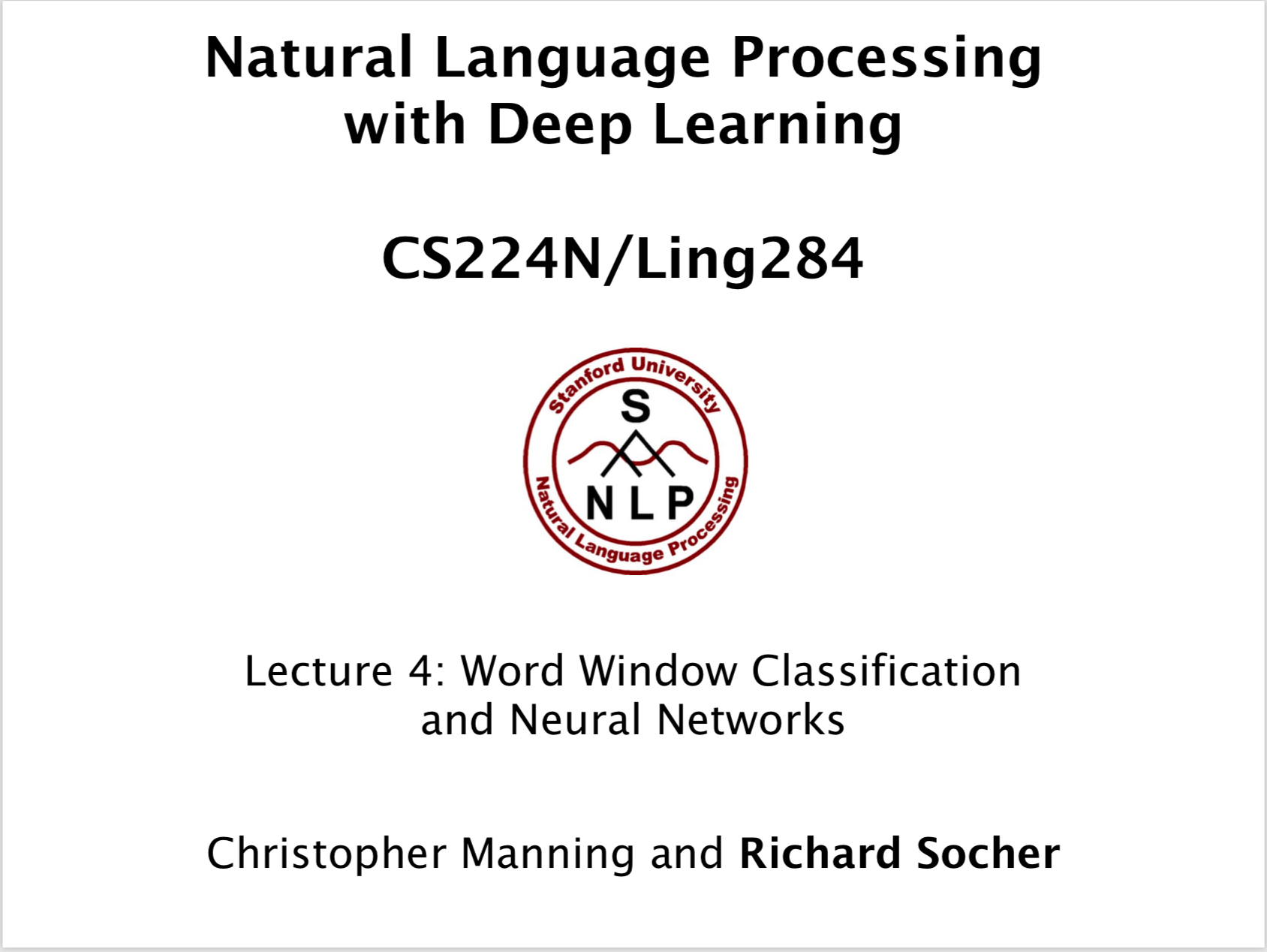## 分类问题 - 逻辑回归的拓展

• ${x^{(i)},y^(i)}^N_{i=1}$
• $x_i$：输入，e.g. words (indices or vectors!), context windows, sentences, documents, etc.
• $y_i$：输入的标签向量
• $\mathcal{R}^{C\ \times\ d}$ ：代表该矩阵是 $C\ \times\ d$的

### The softmax and cross-entropy error

$H(p,q) = H(p) + D_{KL}(p||q)$

The KL divergence is not a distance but a non-symmetric measure of the difference between two probability distributions p and q

$D_{KL}(p||q) = \sum_{c=1}^C p(c)\log{\frac{p(c)}{q(c)}}$

$H(p,q) = -\sum_{c=1}^C p(c)log{q(c)}$

### 一些小建议

1. 在训练集中，训练的向量中telly, TVtelevision 这三个词在一起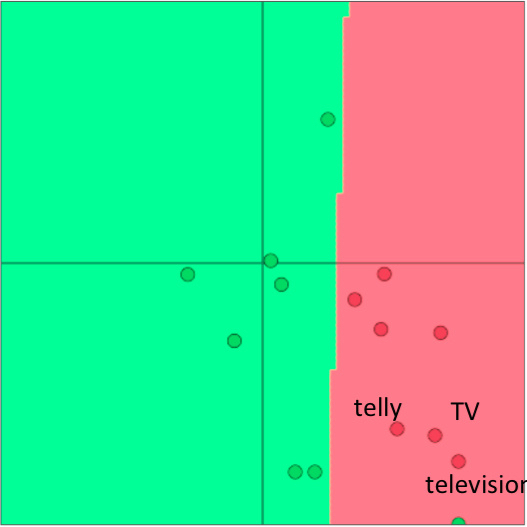2. 如果重新训练，可能 television 没有划分在里面，那么训练结果显然就有问题了。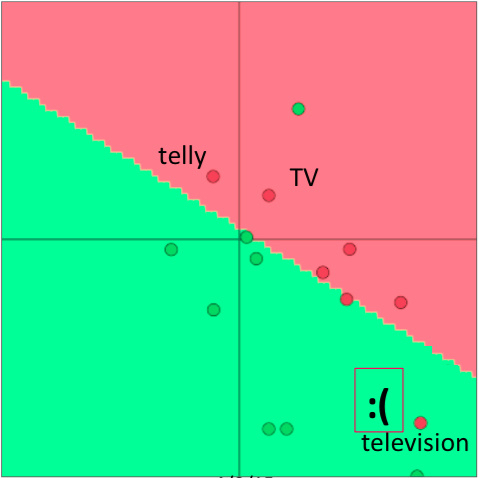之前我们在 softmax 来表示 $p(y|x)$ 中使用的是 $W_y·x$ 这个公式作为 $exp(f(x))$ 中的 $f(x)$ 。

## Window classification

$\hat y = p(y|x) = \frac{exp(W_y·x)}{\sum\nolimits_{c=1}^C exp(W_c·x)}$

### 小建议

1. 小心的定义你的变量们，并且一直跟踪他们的维度
2. 使用链式法则 (Chain Rules)！

## 神经网络

• neuron：个人的理解就是一个逻辑回归连一个激活函数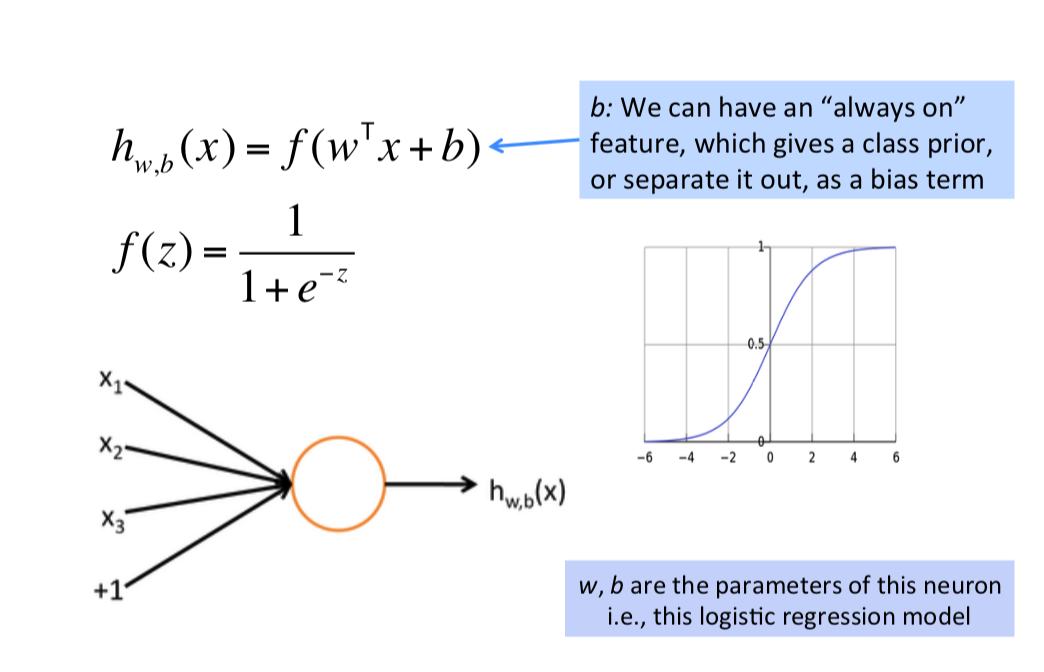• A neural network：多个逻辑回归的输出，有几个逻辑回归输出下一层就有几个神经元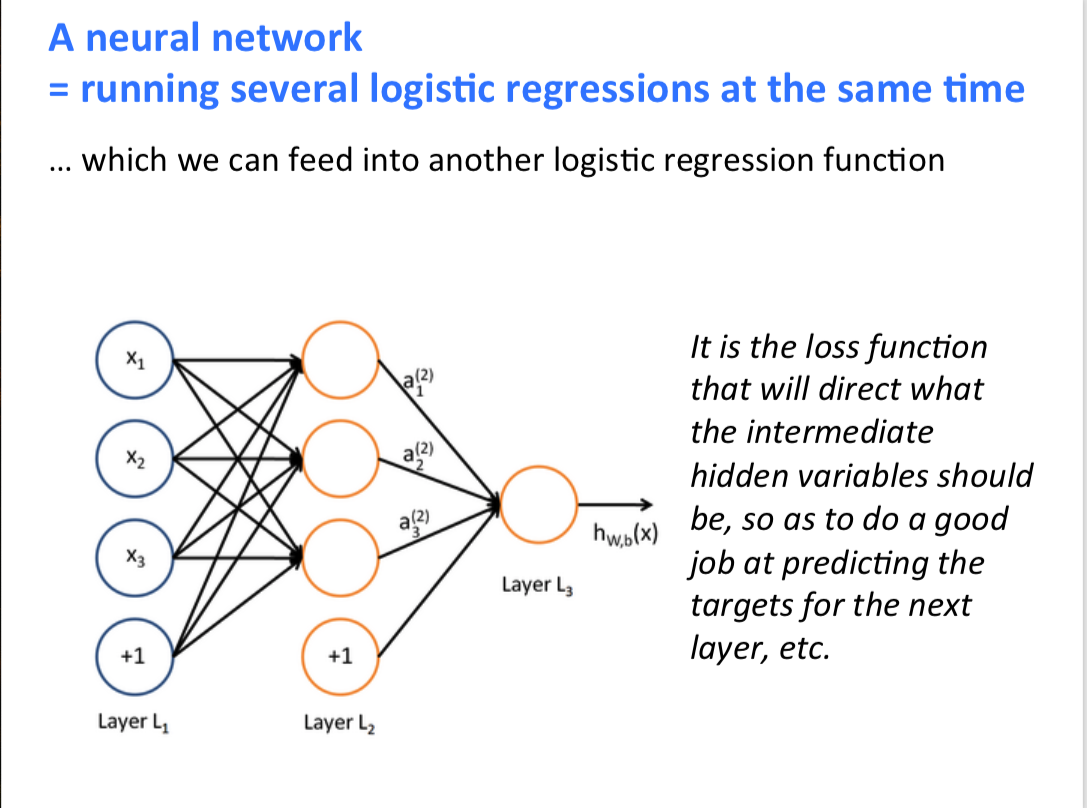• $score(x)$：得分函数，可以理解为前向传播的结果

### 一些矩阵方面的说明可以看到中间层中每一个神经元对应了一个 $W$ 矩阵，图中的一共 3 个 $W$ 。

## 有意思的说明

1. 使用矩阵来运算而不是写个 for 循环单独对一个个向量运算会快很多。帅小哥的例子中快了 12 倍。所以矩阵是你的朋友，所以尽可能多用他们。2. 在实验中神经网络多出来的那一层（隐含层）的作用，可以理解为是不同输入词之间的非线性作用
3. 传统 ML 和神经网络的区别
4. 实验的流程（这一波不知道为什么 PPT 中没给）
• Establish a baseline：选一个模型来训练作为你的基准
• Implement existing neural net model
• Always be close to your data：
1. 可视化他们
2. Collect summart statistics
3. 查看模型错误
4. 注意超参的影响
• Try out different model variants
• 多选用多种分布的数据集进行比对结果，看看哪些分布没有捕捉到
5. 研究的流程
1. 早点开始调研看 paper
2. 拼直觉（本事）找到现有模型的漏洞
3. 和导师聊自己的看法
4. 测试自己的想法（但是要小心验证） 简单的来说就是，大胆猜想，谨慎验证，现在行动
6. SGD 的一些说法：
• 由于使用 SGD，你不停的在随机更新，这使得你的模型不容易陷入局部最优解，这也是SGD比较快，效果还好的一部分原因。
• 事实上你的窗口越小，你的随机性越大
7. 关于 BP 的推导在大佬的文章中有，大家可以自行查看，之后在作业的总结中还会推。